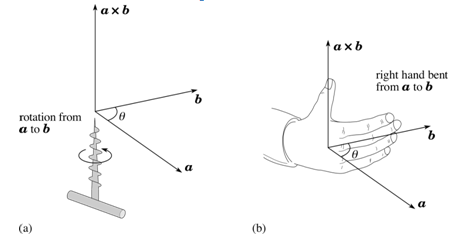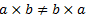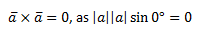Checkout JEE MAINS 2022 Question Paper Analysis : Checkout JEE MAINS 2022 Question Paper Analysis :

# Vector Product Of Two Vectors And Their Properties

The cross product, area product or the vector product of two vectors is a binary operation on two vectors in three-dimensional spaces. It is denoted by ×. The cross product of two vectors is a vector.

Let us consider two vectors denoted as. Let the product (also a vector) of these two vectors be denoted as.

## Magnitude of the vector product

The magnitude of the vector product is given as,Where a and b are the magnitudes of the vector and Ɵ is the angle between these two vectors. From the figure, we can see that there are two angles between any two vectors, that is, Ɵ and (360° – Ɵ). In this rule, we always consider the smaller angle that is less than 180°.

## Direction of the vector productThe right-hand thumb rule is used in which we curl up the fingers of right hand around a line perpendicular to the plane of the vectors a and b and the curl the fingers in the direction from a to b, then the stretched thumb points in the direction of c.

### Commutative property

Unlike the scalar product, cross product of two vectors is not commutative in nature.

Mathematically, for scalar productsBut for vector productsAs we know, the magnitude of both the cross products a × b and b × a is the same and is given by absinθ; but the curling of the right-hand fingers in case of a × b is from a to b, whereas in case of (b × a) it is from b to a, as per which, the two vectors are in opposite directions.

Mathematically,### Distributive property

Like the scalar product, vector product of two vectors is also distributive with respect to vector addition. Mathematically,In order to deal with the vector product of any two vectors, we need to know the vector product of two elementary vectors.Similarly, for the unit vectors following results hold good,